# Machine learning for package users with R (3): Support Vector Machine

Actually support vector machine (SVM) is the one that I love the most among various machine learning classifiers... because of its strong generalization and beautiful decision boundary (in high dimensional space). Although there are other better classifier than SVM, every time I can't help trying it on any data. :)

At any rate, I believe SVM is one of the most widely used machine learning classifier all over the world. It's advantage is: 1) high performance but with a long history, 2) a lot of well-supported libraries or packages of SVM, and 3) highly efficient performance on linearly non-separable patterns.

About 1), I have to emphasize SVM has already more than 20 years history. Can you believe SVM first appeared even before the 1st iPod???*1 There are a plenty of accumulated knowledge about its application and possible problems. 2) is the most important I think because now we have LIBSVM, LIBLINEAR, Kernel Lab, Weka or scikit-learn with implementation of SVM. Some of them can be imported to multiple platforms*2. Finally and needless to say, 3) means its great advantage derived from the kernel method.

### A brief trial on a short version of MNIST datasets

> train<-read.csv("short_prac_train.csv")
> train$label<-as.factor(train$label)
> test$label<-as.factor(test$label)
> library(e1071)
> train.tune<-tune.svm(label~.,data=train,kernel='polynomial',degree=5)
# Polynomial kernel with 5 degrees, chosen in according to Burgess (NIPS, 1996)
> train.tune$best.model Call: best.svm(x = label ~ ., data = train, degree = 5, kernel = "polynomial") Parameters: SVM-Type: C-classification SVM-Kernel: polynomial cost: 1 degree: 5 gamma: 0.00127551 coef.0: 0 Number of Support Vectors: 1808 > train.svm<-svm(label~.,train,cost=train.tune$best.model$cost,gamma=train.tune$best.model$gamma,coef0=train.tune$best.model$coef0,kernel='polynomial',degree=5) > table(test$label,predict(train.svm,newdata=test[,-1]))

0  1  2  3  4  5  6  7  8  9
0 92  3  0  0  0  1  3  1  0  0
1  0 99  0  0  0  0  0  1  0  0
2  2  8 88  0  0  0  0  1  1  0
3  1  6  1 81  0  2  0  0  5  4
4  0  4  0  0 91  0  1  1  1  2
5  2  8  0  5  0 84  1  0  0  0
6  1  5  0  0  0  3 91  0  0  0
7  0  5  0  0  2  0  0 90  1  2
8  0  3  0  1  0  1  0  0 95  0
9  0  4  0  0  1  0  0  3  1 91
> sum(diag(table(test$label,predict(train.svm,newdata=test[,-1]))))/nrow(test)  0.902  Improving the Accuracy and Speed of Support Vector Machines I found Gaussian kernel almost never works so I set hyper parameters according to an article above. This is just a result from the limited part of MNIST datasets so I think accuracy more than 90% would be considerably good. ### Algorithm summary In order to understand what's going on underlying SVM, please just read chapter 12 of the textbook ESL; Its essence is well explained. In short, the essence of SVM can be described by just two keywords. 1. Maximal margin 2. Kernel method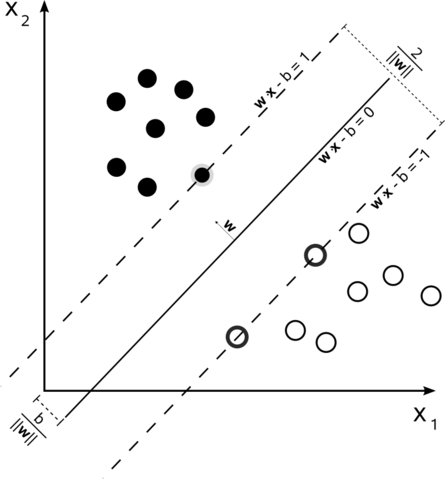Maximal margin is one of the greatest idea for machine learning classifier, I believe. Its robustness and generalization are strongly supported by VC theory and they enable SVM to draw "smooth and beautiful" decision boundaries across data vectors. In order to keep a distance between two classes as far as possible, SVM algorithm only chooses representative data samples as "support vectors" and makes them "sparse"; the algorithm just computes the best decision boundary around the support vectors. This idea leads to well generalized classification because it only considers support vectors, not all data samples. Based on VC theory, the algorithms accepts a minimum range of error rate predicted by the theory that means consequent decision boundary may show some incorrectly classified samples but also result in well generalized classification. Below is a pseudo code of 1-norm soft margin SVM algorithm from Christianini's textbook. In this case, some range of error rate is accepted but the algorithm will give a well generalized decision boundary.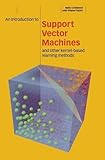An Introduction to Support Vector Machines and Other Kernel-based Learning Methods Given a training sample datasetsand a learning rate,repeat fortoifthenelse ifthenend for until the stop criterion satisfied] returnFinally we get a classification function aswhenis the number of support vectors. A form of this equation shows its decision boundary will be given only by support vectors and this is the most different point from linear models... SVM cannot directly compute feature weights although linear models can easily compute them as coefficients. On the other hand, kernel method is also the most important advantage of SVM ; even a simple linear classification function can classify linearly non-separable patterns with this method. Just as a formula, "kernel" already appears in this post:in the pseudo code above. In the case of usual linear SVM it's merely an inner product, but in the case with kernel method for linearly non-separable patterns kernel function is used. According to its formula, kernel function can be categorized primarily into 4 types*3 below (in particular in the case of svm() function of {e1071} package). Heremeans each data sample point taken at each iteration step andmeans all other samples. • Linear:• Polynomial:• Radial basis fucntion (RBF) or Gaussian:• Sigmoid:Just intuitively, each kernel maps data points to another higher-dimensional space((ESL mentions it in relation to reproducing kernel Hilbert spaces (RKHS))). Let's see an example of RBF kernel. Please download a "double-circle" dataset from here and run as below. > dc<-read.table("double_circle.txt",header=T) > par(mfrow=c(2,2)) > plot(dc[,-3],col=c(rep('blue',300),rep('red',300)),xlim=c(-4,4),ylim=c(-4,4),pch=19,cex=2) > plot(c(),type='n',xlim=c(-4,4),ylim=c(-4,4),axes=F,xlab='',ylab='') > zdc<-rep(0,600) > for (i in 1:600){ + for (j in 1:600){ + zdc[i]<-zdc[i]+exp(-((dc[i,1]-dc[j,1])^2+(dc[i,2]-dc[j,2])^2)) + } + } > library(scatterplot3d) > scatterplot3d(dc$x,dc$y,zdc,color=c(rep('blue',300),rep('red',300)),pch=19,cex.symbols=1.5) > scatterplot3d(dc$x,dc$y,zdc,color=c(rep('blue',300),rep('red',300)),pch=19,cex.symbols=1.5,angle=0)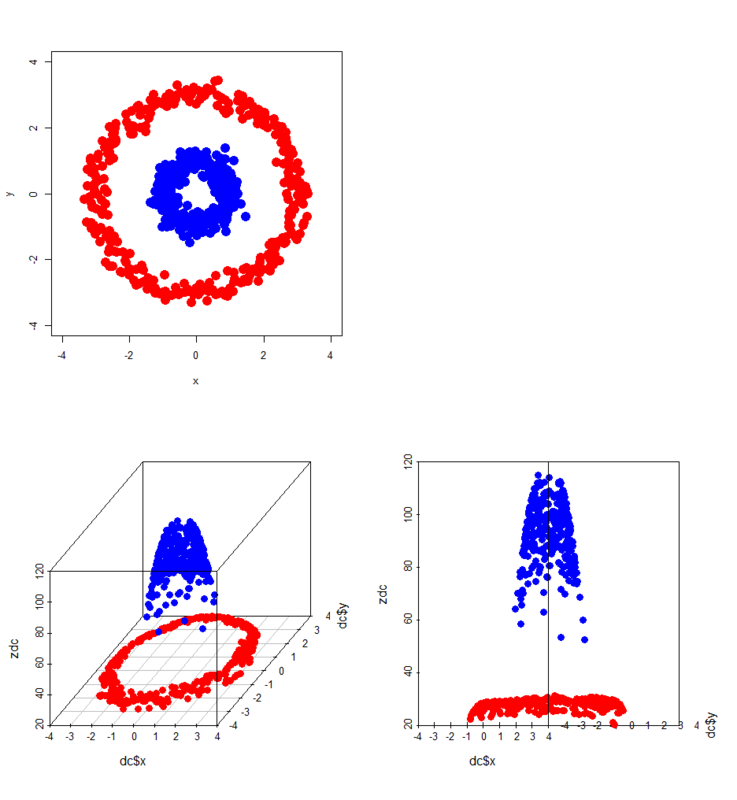A obvious linearly non-separable pattern (top-left) is mapped onto 3D space with RBF kernel. The result clearly shows it can be much easily classified with a simple horizontal plane. Indeed svm() function of {e1071} package classifies it as below. > par(mfrow=c(1,1)) > library(e1071) > dc$label<-as.factor(dc$label) > dc.svm<-svm(label~.,dc) > px<-seq(-4,4,0.03) > py<-seq(-4,4,0.03) > pgrid<-expand.grid(px,py) > names(pgrid)<-names(dc)[-3] > plot(dc[,-3],col=c(rep('blue',300),rep('red',300)),xlim=c(-4,4),ylim=c(-4,4),pch=19,cex=2) > par(new=T) > contour(px,py,array(predict(dc.svm,newdata=pgrid),dim = c(length(px),length(py))),col='purple',lwd=5,levels=0.5,drawlabels=F,xlim=c(-4,4),ylim=c(-4,4))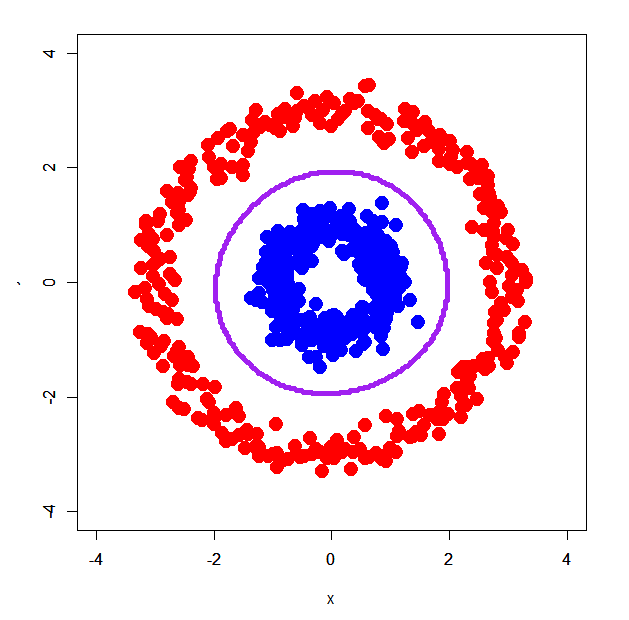Perfectly a decision boundary of SVM classifies two groups of dots. Usually in the case of linearly non-separable patterns RBF kernel or polynomial kernel works well. ### How it works on XOR patterns and linearly separable patterns OK, let's see how SVM works on our good-old XOR patterns and linearly separable patterns. #### XOR patterns Please just run as below with two datasets of XOR patterns. > xors<-read.table("xor_simple.txt",header=T) > xorc<-read.table("xor_complex.txt",header=T) > xors$label<-as.factor(xors$label-1) > xorc$label<-as.factor(xorc$label-1) > xors.svm<-svm(label~.,xors) > xorc.svm<-svm(label~.,xorc) > par(mfrow=c(1,2)) > # Plot for simple XOR > plot(c(),type='n',xlim=c(-4,4),ylim=c(-4,4)) > par(new=T) > rect(0,0,4,4,col='#aaaaff') > par(new=T) > rect(-4,0,0,4,col='#ffaaaa') > par(new=T) > rect(-4,-4,0,0,col='#aaaaff') > par(new=T) > rect(0,-4,4,0,col='#ffaaaa') > par(new=T) > plot(xors[,-3],col=c(rep('blue',50),rep('red',50)),xlim=c(-4,4),ylim=c(-4,4),pch=19,cex=2.5) > par(new=T) > contour(px,py,array(predict(xors.svm,newdata=pgrid),dim = c(length(px),length(py))),levels = 0.5,drawlabels = T,col='purple',lwd=3,xlim=c(-4,4),ylim=c(-4,4)) > # Plot for complex XOR > plot(c(),type='n',xlim=c(-4,4),ylim=c(-4,4)) > par(new=T) > rect(0,0,4,4,col='#aaaaff') > par(new=T) > rect(-4,0,0,4,col='#ffaaaa') > par(new=T) > rect(-4,-4,0,0,col='#aaaaff') > par(new=T) > rect(0,-4,4,0,col='#ffaaaa') > par(new=T) > plot(xorc[,-3],col=c(rep('blue',50),rep('red',50)),xlim=c(-4,4),ylim=c(-4,4),pch=19,cex=2.5) > par(new=T) > contour(px,py,array(predict(xorc.svm,newdata=pgrid),dim = c(length(px),length(py))),levels = 0.5,drawlabels = T,col='purple',lwd=3,xlim=c(-4,4),ylim=c(-4,4))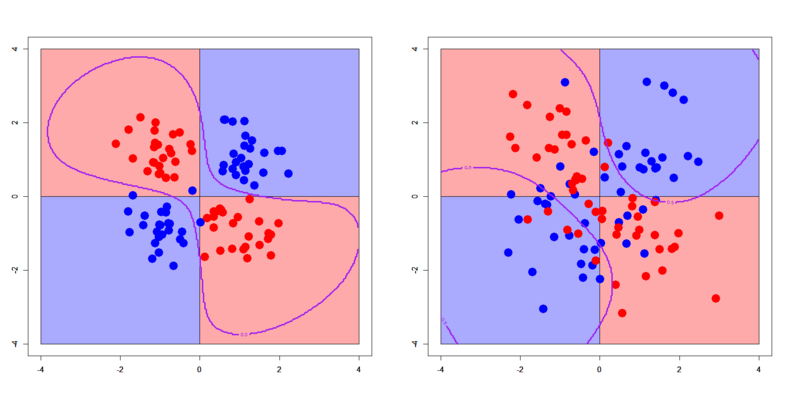These plots show a typical characteristic of soft-margin kernel SVM: well-generalized, with smooth decision boundaries, even with some mis-classified samples. Please remember that of decision trees or logistic regression... you'll find how beautiful the decision boundaries of SVM are! But at the same time you have to be careful about how parameter tuning works on its result. In this case, two hyper parameters play an important role: cost and gamma. Here I tried two patterns of them. > xorc.svm2<-svm(label~.,xorc,cost=10,gamma=10) > xorc.svm3<-svm(label~.,xorc,cost=0.5,gamma=(1/2)/10) # Plot scripts omitted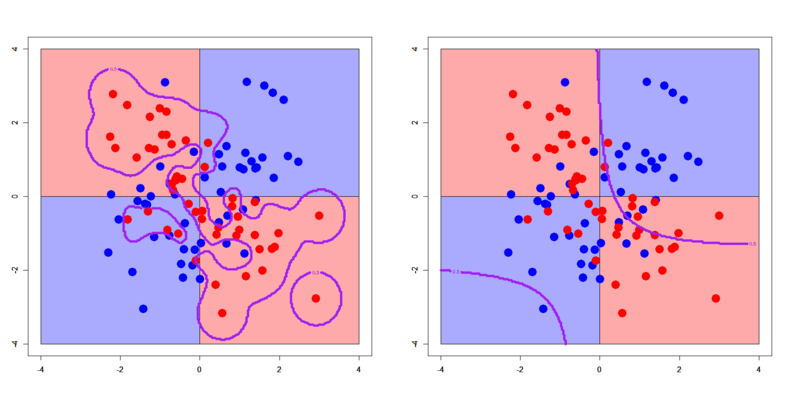For RBF kernel, larger gamma and cost result in less-generalized classification, and less of them result in too-much generalized one. You'll find either of them should not be used. In order to tune parameters and make SVM classifier better, you have to use tune.svm() function. #### Linearly separable patterns In principle SVM can be regarded as an extended version of a linear classifier so that SVM should work also on usual linearly separable patterns. But there are some pitfalls... At any rate, please prepare two datasets of linearly separable patterns and just run as below. > dbi<-read.table("linear_bi.txt",header=T) > dml<-read.table("linear_multi.txt",header=T) > dbi$label<-as.factor(dbi$label) > dml$label<-as.factor(dml\$label)
> dbi.svm2<-svm(label~.,dbi,kernel='linear')
> px2<-seq(-0.5,4.5,0.1)
> py2<-seq(-0.5,4.5,0.1)
> pgrid2<-expand.grid(px2,py2)
> names(pgrid2)<-names(dbi)[-3]
> par(mfrow=c(1,2))
# RBF kernel
> plot(c(),type='n',xlim=c(-0.5,3.5),ylim=c(-0.5,3.5))
> par(new=T)
> polygon(c(-0.5,-0.5,3.5),c(3.5,-0.5,-0.5),col='#aaaaff')
> par(new=T)
> polygon(c(-0.5,3.5,3.5),c(3.5,-0.5,3.5),col='#ffaaaa')
> par(new=T)
> plot(dbi[,-3],pch=19,col=c(rep('blue',25),rep('red',25)),cex=3,xlim=c(-0.5,3.5),ylim=c(-0.5,3.5))
> par(new=T)
> contour(px2,py2,array(predict(dbi.svm1,newdata=pgrid2),dim = c(length(px2),length(py2))),xlim=c(-0.5,3.5),ylim=c(-0.5,3.5),lwd=6,col='purple',levels = 0.5,drawlabels = T)
# Linear kernel
> plot(c(),type='n',xlim=c(-0.5,3.5),ylim=c(-0.5,3.5))
> par(new=T)
> polygon(c(-0.5,-0.5,3.5),c(3.5,-0.5,-0.5),col='#aaaaff')
> par(new=T)
> polygon(c(-0.5,3.5,3.5),c(3.5,-0.5,3.5),col='#ffaaaa')
> par(new=T)
> plot(dbi[,-3],pch=19,col=c(rep('blue',25),rep('red',25)),cex=3,xlim=c(-0.5,3.5),ylim=c(-0.5,3.5))
> par(new=T)
> contour(px2,py2,array(predict(dbi.svm2,newdata=pgrid2),dim = c(length(px2),length(py2))),xlim=c(-0.5,3.5),ylim=c(-0.5,3.5),lwd=6,col='purple',levels = 0.5,drawlabels = T)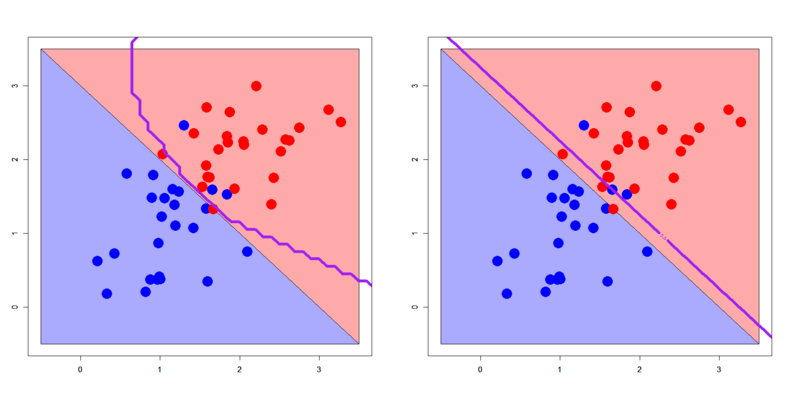How do you feel a result in the left panel? Compared to the right one? Yes, in this case RBF kernel gives less-generalized classifier. 3-classes pattern shows it more clearly.

> dml.svm1<-svm(label~.,dml,kernel='radial')
> dml.svm2<-svm(label~.,dml,kernel='linear')
> par(mfrow=c(1,2))
> plot(c(),type='n',xlim=c(-0.5,4.5),ylim=c(-0.5,4.5))
> par(new=T)
> polygon(c(-0.5,-0.5,3.5),c(3.5,-0.5,-0.5),col='#aaaaff')
> par(new=T)
> polygon(c(-0.5,3.5,4.5,4.5,1.0,-0.5),c(3.5,-0.5,-0.5,1.0,4.5,4.5),col='#ffaaaa')
> par(new=T)
> polygon(c(1.0,4.5,4.5),c(4.5,1.0,4.5),col='#ccffcc')
> par(new=T)
> plot(dml[,-3],pch=19,col=c(rep('blue',25),rep('red',25),rep('green',25)),cex=3,xlim=c(-0.5,4.5),ylim=c(-0.5,4.5))
> par(new=T)
> contour(px2,py2,array(predict(dml.svm1,newdata=pgrid2),dim=c(length(px2),length(py2))),xlim=c(-0.5,4.5),ylim=c(-0.5,4.5),col="purple",lwd=6,drawlabels=T,levels=c(0.5,1.5))
> plot(c(),type='n',xlim=c(-0.5,4.5),ylim=c(-0.5,4.5))
> par(new=T)
> polygon(c(-0.5,-0.5,3.5),c(3.5,-0.5,-0.5),col='#aaaaff')
> par(new=T)
> polygon(c(-0.5,3.5,4.5,4.5,1.0,-0.5),c(3.5,-0.5,-0.5,1.0,4.5,4.5),col='#ffaaaa')
> par(new=T)
> polygon(c(1.0,4.5,4.5),c(4.5,1.0,4.5),col='#ccffcc')
> par(new=T)
> plot(dml[,-3],pch=19,col=c(rep('blue',25),rep('red',25),rep('green',25)),cex=3,xlim=c(-0.5,4.5),ylim=c(-0.5,4.5))
> par(new=T)
> contour(px2,py2,array(predict(dml.svm2,newdata=pgrid2),dim=c(length(px2),length(py2))),xlim=c(-0.5,4.5),ylim=c(-0.5,4.5),col="purple",lwd=6,drawlabels=T,levels=c(0.5,1.5))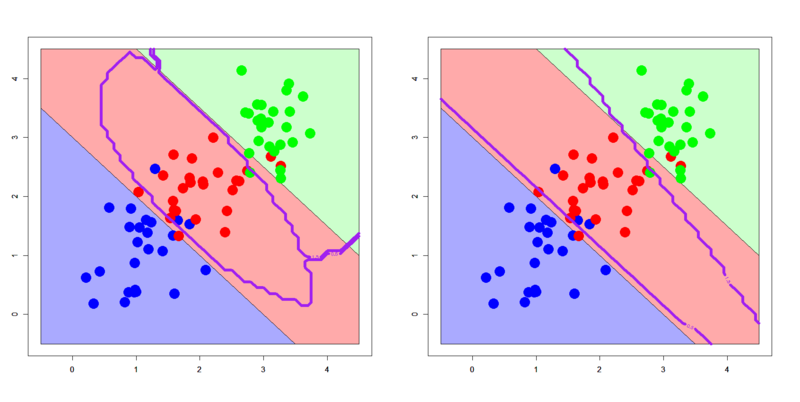RBF kernel fails to draw its decision boundary well along to the assumed true boundaries, although linear kernel is successful. Choosing which kernel is very important.

### Conclusions

Lessons in this post are:

1. SVM is a strong method for linearly non-separable patterns
2. But it cannot work ideally without fine parameter tuning
3. You have to also pay attention to which kernel should be used to what kind of datasets

By the way, results of SVM in this post were produced by svm() function of {e1071} package, that is a binding for R of famous LIBSVM library.

LIBSVM is a cross-platform library so that it works in the (almost) same manner across R, Java, Python, Ruby, C#, Matlab, Haskell, Lisp, or even PHP. You can get almost the same result from e.g. Java frameworks as that from R.

*1:SVM got to be widely used after Platt's excellent work in 1999, but the 1st iPod was released in 2001

*2:LIBSVM can be used with R as {e1071}, Python, Java, Matlab, C or C++

*3:Christianini's textbook suggests the other kinds of kernel function, for example, a sophisticated kernel for a specific use - text mining